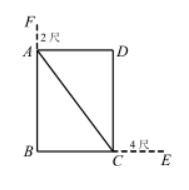$(x-4)^2+(x-2)^2=x^2$

$\because x>4, \therefore$ 只敢 $x=10$,